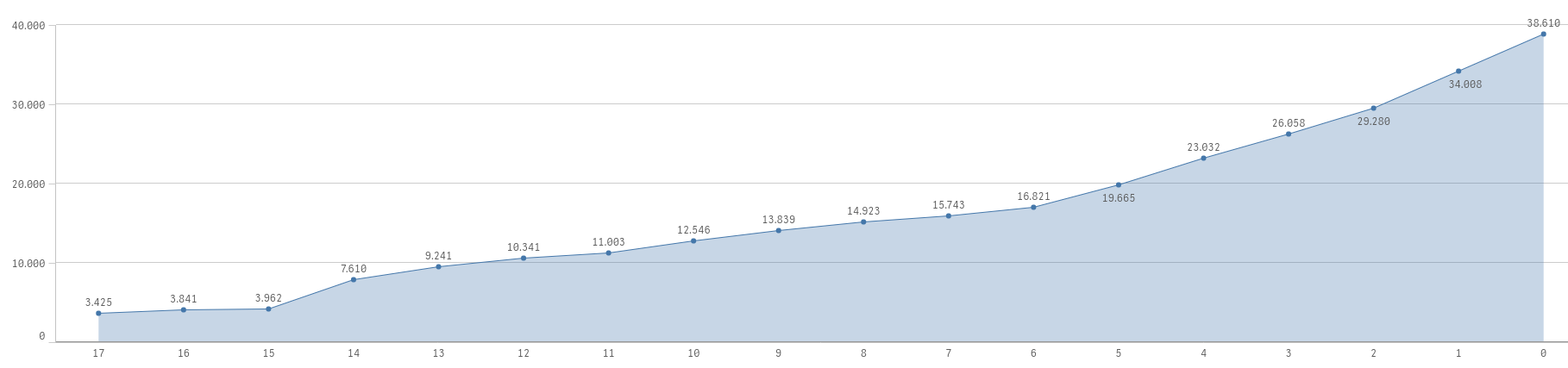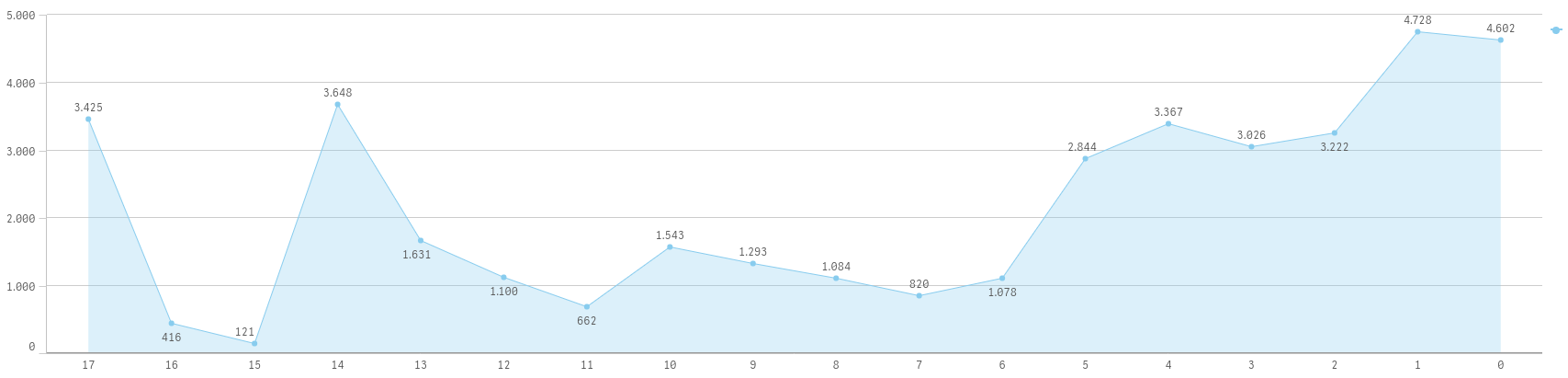# Qlik Sense App Development

Discussion board where members can learn more about Qlik Sense App Development and Usage.

Highlighted
New Contributor II

## Cumulative Sum

Hello,

I try to make a cumulative graph, I use: Rangesum( Above(Sum(quantity), 0, RowNo())) everything works fine.But When I try to add a new dimension I have a problem:Also I try to use: Aggr(Rangesum( Above(Sum(quantity), 0, RowNo())), week)

I want to make graph to be like the 1st graph:

Dimenstion are: week, name

Measure are: quantity

Thanks

Tags (3)
1 Solution

Accepted Solutions
New Contributor II

## Re: Cumulative Sum

I solve with: Aggr(Rangesum(Above(Sum(quantity), 0, RowNo())), name, (week, (NUMERIC, DESCENDING)))

1 Reply
New Contributor II

## Re: Cumulative Sum

I solve with: Aggr(Rangesum(Above(Sum(quantity), 0, RowNo())), name, (week, (NUMERIC, DESCENDING)))